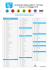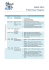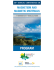# Formula Sheet for LSU Physics 2113, Third Exam, Fall ’14

## Transcription

Formula Sheet for LSU Physics 2113, Third Exam, Fall ’14
```Formula Sheet for LSU Physics 2113, Third Exam, Fall ’14
• Constants, definitions:
m
g = 9.8 2
s
m3
G = 6.67 × 10−11
kg · s2
MSun = 1.99 × 1030 kg
C2
o = 8.85 × 10−12
Nm2
8
c = 3.00 × 10 m/s
REarth = 6.37 × 106 m
MEarth = 5.98 × 1024 kg
RM oon = 1.74 × 106 m
Earth-Sun distance = 1.50×1011 m
MM oon = 7.36 × 1022 kg
1
Nm2
= 8.99 ×109 2
k=
C
4πo
mp = 1.67 × 10−27 kg
Earth-Moon distance = 3.82×108 m
dipole moment: p
~ = q d~
me = 9.11 × 10−31 kg
Area of a circle: A = πr 2
Area of a cylinder: A = 2πr`
Area of a sphere: A = 4πr 2
Volume of a cylinder: V = πr 2 `
e = 1.60 × 10−19 C
1 eV = e(1V) = 1.60×10−19 J
Q
Q
Q
charge densities: λ = , σ = , ρ =
L
A
V
Volume of a sphere: V = 43 πr 3
• Units:
Joule = J = N·m
• Kinematics (constant acceleration):
v = vo + at
x − xo = 21 (vo + v)t
• Circular motion:
mv 2
Fc = mac =
,
r
T =
2πr
v
,
x − xo = vo t + 21 at2
v 2 = vo2 + 2a(x − xo )
v = ωr
• General (work, def. of potential energy, kinetic energy):
~net = m~
K = 12 mv 2
F
a
Emech = K + U
W = −∆U (by field) Wext = ∆U = −W (if objects are initially and finally at rest)
• Gravity:
~| = G
Newton’s law: |F
m1 m2
r2
Gravitational Field: ~
g = −G
Law of periods:
T2
4π 2
=
GM
M
r2
!
rˆ = −
r
dVg
dr
3
Potential Energy of a System (more than 2 masses):
I
GM
Gravitational acceleration (planet of mass M ): ag =
r2
GM
Gravitational potential: Vg = −
r
m1 m2
Potential Energy: U = −G
r12
m1 m2
m1 m3
m2 m3
U =− G
+G
+G
+ ...
r12
r13
r23
~ = −4πGMins
~
g · dS
Gauss’ law for gravity:
S
• Electrostatics:
~| = k
Coulomb’s law: |F
| q1 || q2 |
~ = qE
~
Force on a charge in an electric field: F
r2
|q|
~ =k
Electric field of a point charge: |E|
r2
2k~
p
~ =
Electric field of a dipole on axis, far away from dipole: E
z3
2kλ
~ =
Electric field of an infinite line charge: |E|
r
~
Torque on a dipole in an electric field: ~
τ =p
~×E
~
~
Potential energy of a dipole in E field: U = −~
p·E
• Electric flux: Φ =
R
~ · dA
~
E
• Gauss’ law: o
I
~ · dA
~ = qenc
E
• Electric field of an infinite non-conducting plane with a charge density σ: E =
σ
2o
• Electric field of infinite conducting plane or close to the surface of a conductor: E =
σ
o
• Electric potential, potential energy, and work:
Vf − Vi = −
Z f
~ · d~
E
s
i
~
~ = −5V,
E
Ex = −
~ · ∆~
In a uniform field: ∆V = −E
s = −Ed cos θ
∂V
∂x
, Ey = −
∂V
, Ez = −
∂V
∂y
∂z
n
n
X
X
qi
q
Potential of n point charges: V =
Vi = k
Potential of a point charge q: V = k
r
r
i=1
i=1 i
Electric potential energy: ∆U = q∆V ∆U = −Wfield
q1 q2
Potential energy of two point charges: U12 = Wext = q2 V1 = q1 V2 = k
r12
• Capacitance: definition: q = CV
Capacitor with a dielectric: C = κCair
Potential Energy in Cap: U =
Capacitors in parallel: Ceq
• Current: i =
dq
dt
Z
=
q2
2C
P
= Ci
=
1
2
Parallel plate: C = ε◦
qV =
1
2
CV 2
~ Const. curr. density: J =
J~ · dA,
• Definition of resistance: R =
V
i
L
A
i
A
, Charge carrier0 s drift speed: ~
vd =
J~
ne
~
|E|
|J~|
Temperature dependence: ρ − ρ◦ = ρ◦ α(T − T◦ )
Power dissipated in a resistor: P = i2 R =
• Power in an electrical device: P = iV
V2
R
dW
dq
• Resistors in series: Req =
P
Ri
d
~ 2
Energy density of electric field: u = κεo |E|
2
X 1
1
Capacitors in series:
=
Ceq
Ci
Definition of resistivity: ρ =
• Resistance in a conducting wire: R = ρ
• Definition of emf : E =
1
A
Resistors in parallel:
1
Req
=
X 1
Ri
• Loop rule in DC circuits: the sum of changes in potential across any closed loop of a circuit must be zero.
• Junction rule in DC circuits: the sum of currents entering any junction must be equal to the sum of
currents leaving that junction.
t
t
• RC circuit: Charging: q(t) = CE(1 − e− τc ), Time constant τC = RC, Discharging: q(t) = qo e− τc
• Magnetic Fields:
~ = q~
~
Magnetic force on a charge q: F
v×B
i
Hall voltage: V = vd Bd =
B
d = width ⊥ to field and i,
nle
~ = qE
~ + q~
~
Lorentz force: F
v×B
l = thickness k to field and ⊥ to i
Circular motion in a magnetic field: qv⊥ B =
2
mv⊥
with period: T =
r
~ = iL
~ ×B
~
Magnetic force on a length of wire: F
~
Magnetic Dipole: µ
~ = N iA
~
Torque: ~
τ =µ
~ ×B
(µ0 = 4π × 10−7
• Generating Magnetic Fields:
4π
~
Potential energy: U = −~
µ·B
T·m
)
A
r3
Magnetic field of a long straight wire: B =
µ0 2i
4π r
Magnetic field of a circular arc: B =
Force between parallel current carrying wires: Fab =
I
qB
µ0 id~
s×~
r
~ =
Biot-Savart Law: dB
Ampere’s law:
2πm
~ · d~
B
s = µ0 ienc
Magnetic field of a toroid: B =
µ0 ia ib
2πd
µ0 i
4π r
φ
L
Magnetic field of a solenoid: B = µ0 in
µ0 iN
2πr
~ =
, Magnetic field of a dipole on axis, far away: B
µ0 µ
~
2π z 3
• Induction:
Z
~ · dA
~
B
Magnetic Flux: Φ =
dΦ
dt
I
Induced Electric Field:
(= −N
dΦ
dt
~ · d~
E
s=−
Definition of Self-Inductance: L =
dΦ
dt
NΦ
Inductance of a solenoid: L = µ0 n2 Al
i
EMF (Voltage) across an inductor: E = −L
RL Circuit: Rise of current: i =
Magnetic Energy: UB =
1
2
Li2
E
R
Motional emf: E = BLv
for a coil with N turns)
tR
di
dt
(1 − e− L ), Time constant: τL =
L
R
tR
, Decay of current: i = i0 e− L
Magnetic energy density: uB =
B2
2µ0
```

### ch29_Magnetic_Induct..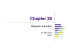### BUSINESS PLAN - Unimatica Confapivarese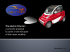### THETA - CROMA - GAMMA - KAPPA - OMEGA - SIGMA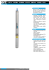### Product Measurement Data Sheet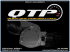### Lecture 13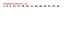### cop. sps 2015 blu 301C - loghi.indd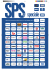### CATALOGO GENERALE ombre:Layout 1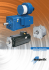### Dipartimento di Lingue e Letterature Straniere Dipartimento di Scienze Giuridiche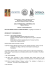### sensori magnetici serie smc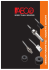### Applications of Magnetism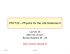### Repertorio Merceologico